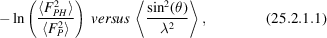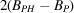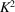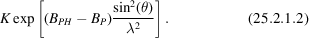International
Tables for
Crystallography
Volume F
Crystallography of biological macromolecules
Edited by M. G. Rossmann and E. Arnold

International Tables for Crystallography (2006). Vol. F, ch. 25.2, p. 696

## Section 25.2.1.3.1. Relative Wilson scaling

W. Fureya*

#### 25.2.1.3.1. Relative Wilson scaling

| top | pdf |

With this method, the derivative scattering, on average, is made equal to the native scattering by plottingwith the averages taken in corresponding resolution shells. A least-squares fit of a straight line to the plot yields a slope equal to(twice the difference between overall isotropic temperature parameters for derivative and native data sets) and an intercept of ln. From these values, the derivative data are put on the scale of the native by multiplying each derivative amplitude by